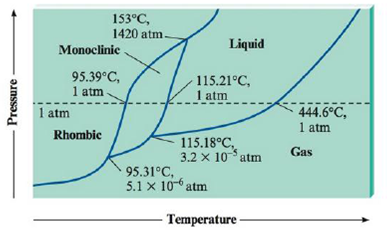# Sulfur exhibits two solid phases, rhombic and monoclinic. Use the accompanying phase diagram for sulfur to answer the following questions. (The phase diagram is not to scale.) a. How many triple points are in the phase diagram? b. What phases are in equilibrium at each of the triple points? c. What is the stable phase at 1 atm and 100.°C? d. What are the normal melting point and the normal boiling point of sulfur? e. Which is the densest phase? f. At a pressure of 1.0 × 10 -5 atm, can rhombic sulfur sublime? g. What phase changes occur when the pressure on a sample of sulfur at 100.°C is increased from 1.0 × 10 −8 atm to 1500 atm?### Chemistry: An Atoms First Approach

2nd Edition
Steven S. Zumdahl + 1 other
Publisher: Cengage Learning
ISBN: 9781305079243

#### Solutions

Chapter
Section### Chemistry: An Atoms First Approach

2nd Edition
Steven S. Zumdahl + 1 other
Publisher: Cengage Learning
ISBN: 9781305079243
Chapter 9, Problem 104E
Textbook Problem
3288 views

## Sulfur exhibits two solid phases, rhombic and monoclinic. Use the accompanying phase diagram for sulfur to answer the following questions. (The phase diagram is not to scale.)a. How many triple points are in the phase diagram?b. What phases are in equilibrium at each of the triple points?c. What is the stable phase at 1 atm and 100.°C?d. What are the normal melting point and the normal boiling point of sulfur?e. Which is the densest phase?f. At a pressure of 1.0 × 10-5 atm, can rhombic sulfur sublime?g. What phase changes occur when the pressure on a sample of sulfur at 100.°C is increased from 1.0 × 10−8 atm to 1500 atm?

Interpretation Introduction

Interpretation: The following statements to be explained by using given phase diagram of Sulphur.

Concept Introduction

Phase diagram: Under different temperature and pressure, physical states of the substance that are represented in graph are known as phase diagram. The temperature represents in X-axis and pressure in Y-axis.

Triple point: For given substance, three phases (solid, liquid and gas) are coexisting in thermodynamic equilibrium at particular temperature and pressure.

Critical point: In a phase diagram, a point at which both liquid and gaseous state of the substance having indistinguishable density.

Normal melting point: Normal melting point of the solids defined as the temperature at which solid melts at 1 atmospheric pressure. Normal melting point is differing from melting point in which pressure would be defined.

Normal boiling point: Normal boiling point of the liquid is defined as the temperature at which liquid boils at 1 atmospheric pressure. Normal boiling point is differing from boiling point in which pressure would be defined.

### Explanation of Solution

Explanation

a)

Record the given data

The given phase diagram for Sulphur was recorded as shown.

Three triple points are present in phase diagram. These are,

1) Rhombic, monoclinic and gas

2) Monoclinic, liquid and gas

3) Monoclinic, liquid and rhombic

b)

In the given phase diagram of Sulphur, in which the triple points at the temperature of 95.31°C are Rhombic, monoclinic and gas. At 115.18°C are monoclinic, liquid and gas and at 153°C are monoclinic, liquid and rhombic.

c)

From phase diagram Monoclinic solid phase is stable point at 100°C and 1atm.

d)

The normal melting point of Sulphur is 115.21°C because which is appears in liquid phase at I atm and normal boiling point of Sulphur is 444.6°C because it is appears in gas phase at 1 atm.

e)

From phase diagram Rhombic is the densest phase, because the monoclinic-rhombic equilibrium line has a positive slope, and the solid-liquid equilibrium lines also has positive slopes.

f)

Rhombic sulphur cannot sublime at pressure of 1.0×105. P = 1.0 × 10-5 atm is at a pressure  between 95

### Still sussing out bartleby?

Check out a sample textbook solution.

See a sample solution

#### The Solution to Your Study Problems

Bartleby provides explanations to thousands of textbook problems written by our experts, many with advanced degrees!

Get Started

Find more solutions based on key concepts
A benefit to health is seen when is used in place of in the diet. a. saturated fat/mono unsaturated fat b. satu...

Nutrition: Concepts and Controversies - Standalone book (MindTap Course List)

Name three carbohydrates that can be built using only glucose monomers.

Biology: The Unity and Diversity of Life (MindTap Course List)

How is sensory information integrated?

Biology (MindTap Course List)

What is the hybridization of nitrogen in

Chemistry: Principles and Reactions

What is the most common type of star?

Horizons: Exploring the Universe (MindTap Course List)

What are the three most common chronic conditions affecting women over age 65?

Nutrition Through the Life Cycle (MindTap Course List)

Two particles with masses m and 3m are moving toward each other along the x axis with the same initial speeds i...

Physics for Scientists and Engineers, Technology Update (No access codes included)

Why did Newton conclude that gravity has to be mutual and universal?

Foundations of Astronomy (MindTap Course List)

Are there tides in the open ocean?

Oceanography: An Invitation To Marine Science, Loose-leaf Versin